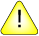This version of this document is no longer maintained. For the latest documentation, see http://www.qnx.com/developers/docs.

# difftime()

Calculate the difference between two times

## Synopsis:

```#include <time.h>

double difftime( time_t time1,
time_t time0 );```

## Arguments:

time1, time0
The times to compare, expressed as time_t objects.

## Library:

libc

Use the -l c option to qcc to link against this library. This library is usually included automatically.

## Description:

The difftime() function calculates the difference between the calendar times specified by time1 and time0:

time1 - time0

## Returns:

The difference between the two times (in seconds).

## Examples:

```#include <stdio.h>
#include <time.h>
#include <stdlib.h>

void compute( void )
{
int i, j;

for( i = 1; i <= 20; i++ ) {
for( j = 1; j <= 20; j++ ) {
printf( "%3d ", i * j );
}
printf( "\n" );
}
}

int main( void )
{
time_t start_time, end_time;

start_time = time( NULL );
compute();
end_time = time( NULL );
printf( "Elapsed time: %f seconds\n",
difftime( end_time, start_time ) );

return EXIT_SUCCESS;
}```

## Classification:

Safety:
Cancellation point No
Interrupt handler No
Signal handler No